$$\require{cancel}$$

# 29A: Waves: Characteristics, Types, Energy

Consider a long taut horizontal string of great length. Suppose one end is in the hand of a person and the other is fixed to an immobile object. Now suppose that the person moves her hand up and down. The person causes her hand, and her end of the string, to oscillate up and down. To discuss what happens, we, in our mind, consider the string to consist of a large number of very short string segments. It is important to keep in mind that the force of tension of a string segment exerted on any object, including another segment of the string, is directed away from the object along the string segment that is exerting the force. (The following discussion and diagrams are intentionally oversimplified. The discussion does correctly give the gross idea of how oscillations at one end of a taut string can cause a pattern to move along the length of the string despite the fact that the individual bits of string are essentially doing nothing more than moving up and down.

The person is holding one end of the first segment. She first moves her hand upward.This tilts the first segment so that the force of tension that it is exerting on the second segment has an upward component.This, in turn, tilts the second segment so that its force of tension on the third segment now has an upward component. The process continues with the 3rd segment, the 4th segment, etc.After reaching the top of the oscillation, the person starts moving her hand downward. She moves the left end of the first segment downward, but by this time, the first four segments have an upward velocity. Due to their inertia, they continue to move upward. The downward pull of the first segment on the left end of the second segment causes it to slow down, come to rest,and eventually start moving downward. Inertia plays a huge role in wave propagation. “To propagate” means “to go” or “to travel.” Waves propagate through a medium.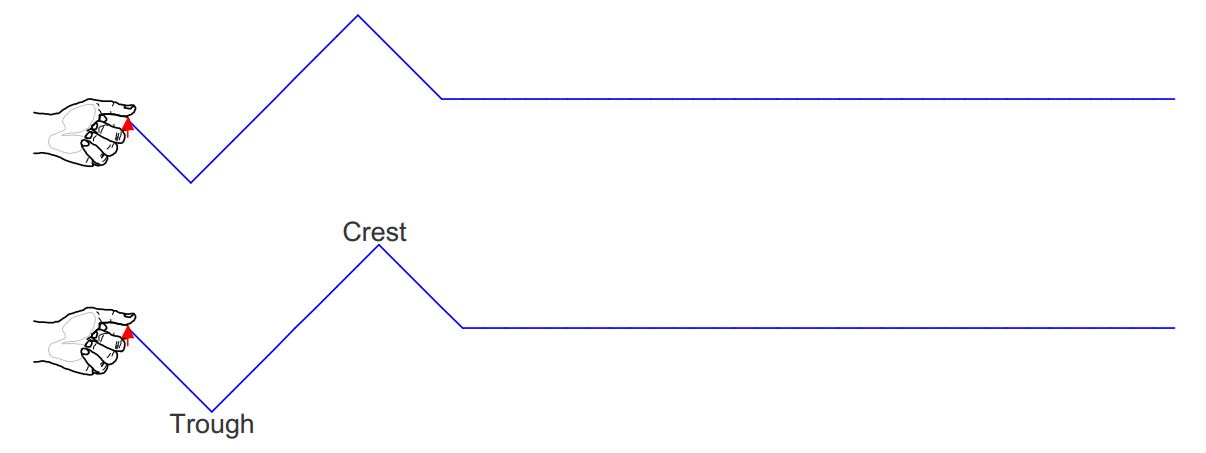Each very short segment of the string undergoes oscillatory motion like that of the hand, but for any given section, the motion is delayed relative to the motion of the neighboring segment that is closer to the hand. The net effect of all these string segments oscillating up and down, each with the same frequency but slightly out of synchronization with its nearest neighbor, is to create a disturbance in the string. Without the disturbance, the string would just remain on the original horizontal line. The disturbance moves along the length of the string, away from the hand. The disturbance is called a wave. An observer, looking at the string from the side sees crests and troughs of the disturbance, moving along the length of the string, away from the hand. Despite appearances, no material is moving along the length of the string, just a disturbance. The illusion that actual material is moving along the string can be explained by the timing with which the individual segments move up and down, each about its own equilibrium position, the position it was in before the person started making waves.

# Wave Characteristics

In our pictorial model above, we depicted a hand that was oscillating but not undergoing simple harmonic motion. If the oscillations that are causing the wave do conform to simple harmonic motion, then each string segment making up the string will experience simple harmonic motion (up and down). When individual segments making up the string are each undergoing simple harmonic motion, the wave pattern is said to vary sinusoidally in both time and space. We can tell that it varies sinusoidally in space because a graph of the displacement $$y$$, the distance that a given point on the string is above its equilibrium position, versus $$x$$, how far from the end of the string the point on the string is; for all points on the string; is sinusoidal.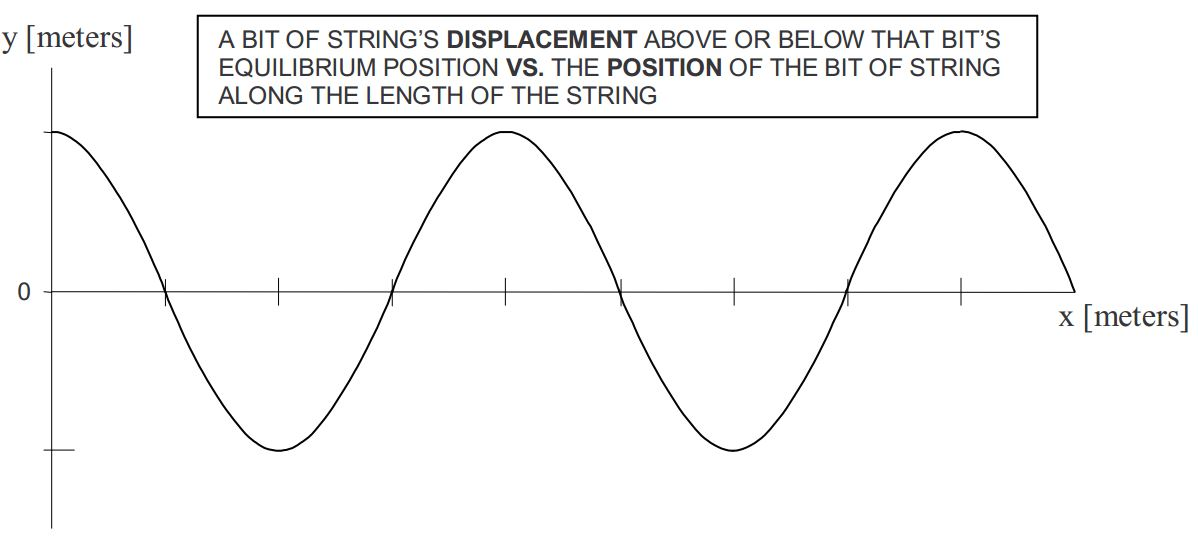We say that the wave varies sinusoidally with time because, for any point along the length of the string, a graph of the displacement of that point from its equilibrium position vs. time is sinusoidal:There are a number of ways of characterizing the wave-on-a-string system. You could probably come up with a rather complete list yourself: the rate at which the oscillations are occurring, how long it takes for a given tiny segment of the string to complete one oscillation, how big the oscillations are, the smallest length of the unique pattern which repeats itself in space, and the speed at which the wave pattern travels along the length of the string. Physicists have, of course, given names to the various quantities, in accordance with that important lowest level of scientific activity—naming and categorizing the various characteristics of that aspect of the natural world which is under study. Here are the names:

# Amplitude

Any particle of a string with waves traveling through it undergoes oscillations. Such a particle goes away from its equilibrium position until it reaches its maximum displacement from its equilibrium position. Then it heads back toward its equilibrium position and then passes right through the equilibrium position on its way to its maximum displacement from equilibrium on the other side of its equilibrium position. Then it heads back toward the equilibrium position and passes through it again. This motion repeats itself continually as long as the waves are traveling through the location of the particle of the string in question. The maximum displacement of any particle along the length of the string, from that point’s equilibrium position, is called the amplitude $$y_{\max}$$ of the wave.

The amplitude can be annotated on both of the two kinds of graphs given above (Displacement vs. Position, and Displacement vs. Time). Here we annotate it on the Displacement vs. Position graph: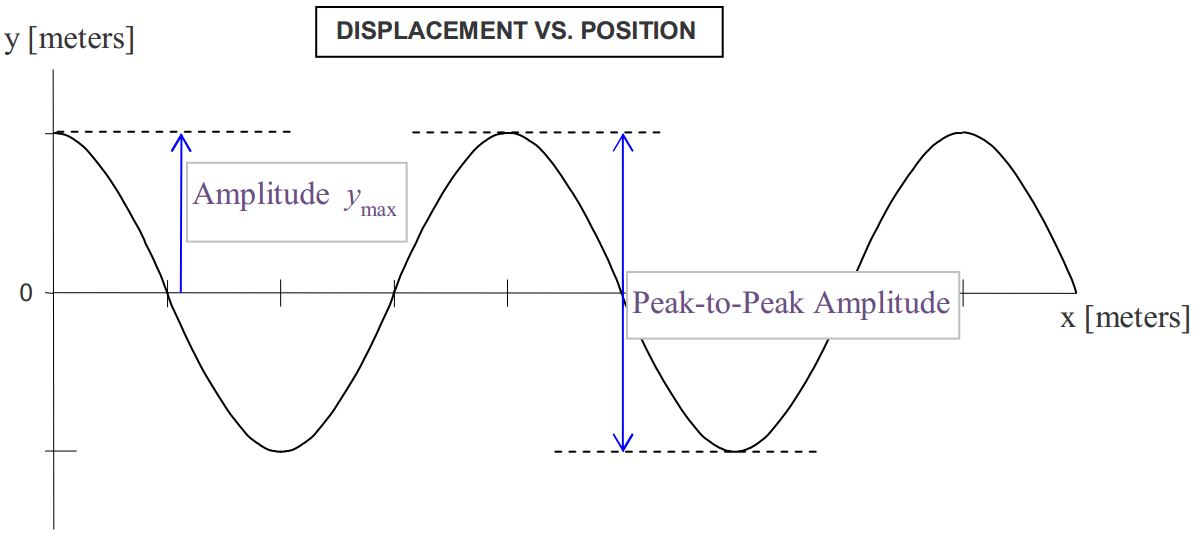The peak-to-peak amplitude, a quantity that is often easier to measure than the amplitude itself, has also been annotated on the graph. It should be obvious that the peak-to-peak amplitude is twice the amplitude.

# Period

The amount of time that it takes any one particle along the length of the string to complete one oscillation is called the period $$T$$. Note that the period is completely determined by the source of the waves. The time it takes for the source of the waves to complete one oscillation is equal to the time it takes for any particle of the string to complete one oscillation. That time is the period of the wave. The period, being an amount of time, can only be annotated on the Displacement vs. Time graph (not on the Displacement vs. Position Along the String graph).# Frequency

The frequency $$f$$ is the number-of-oscillations-per-second that any particle along the length of the string undergoes. It is the oscillation rate. Since it is the number-of-oscillations-per-second and the period is the number-of-seconds-per-oscillation, the frequency $$f$$ is simply the reciprocal of the period $$T$$: $$f=\frac{1}{T}$$.

Amplitude, period, and frequency are quantities that you learned about in your study of oscillations. Here, they characterize the oscillations of a point on a string. Despite the fact that the string as a whole is undergoing wave motion, the fact that the point itself, any point along the length of the string, is simply oscillating, means that the definitions of amplitude, period, and frequency are the same as the definitions given in the chapter on oscillations. Thus, our discussion of amplitude, period, and frequency represents a review. Now, however, it is time to move on to something new, a quantity that does not apply to simple harmonic motion but does apply to waves.

# Wavelength

The distance over which the wave pattern repeats itself once, is called the wavelength $$\lambda$$ of the wave. Because the wavelength is a distance measured along the length of the string, it can be annotated on the Displacement vs. Position Along the String graph (but not on the Displacement vs. Time graph):# Wave Velocity

The wave velocity is the speed and direction with which the wave pattern is traveling. (It is NOT the speed with which the particles making up the string are traveling in their up and down motion.) The direction part is straightforward, the wave propagates along the length of the string, away from the cause (something oscillating) of the wave. The wave speed (the constant speed with which the wave propagates) can be expressed in terms of other quantities that we have just discussed.

To get at the wave speed, what we need to do is to correlate the up-and-down motion of a point on the string, with the motion of the wave pattern moving along the string. Consider the following Displacement vs. Position graph for a wave traveling to the right. In the diagram, I have shaded in one cycle of the wave, marked off a distance of one wavelength, and drawn a dot at a point on the string whose motion we shall keep track of.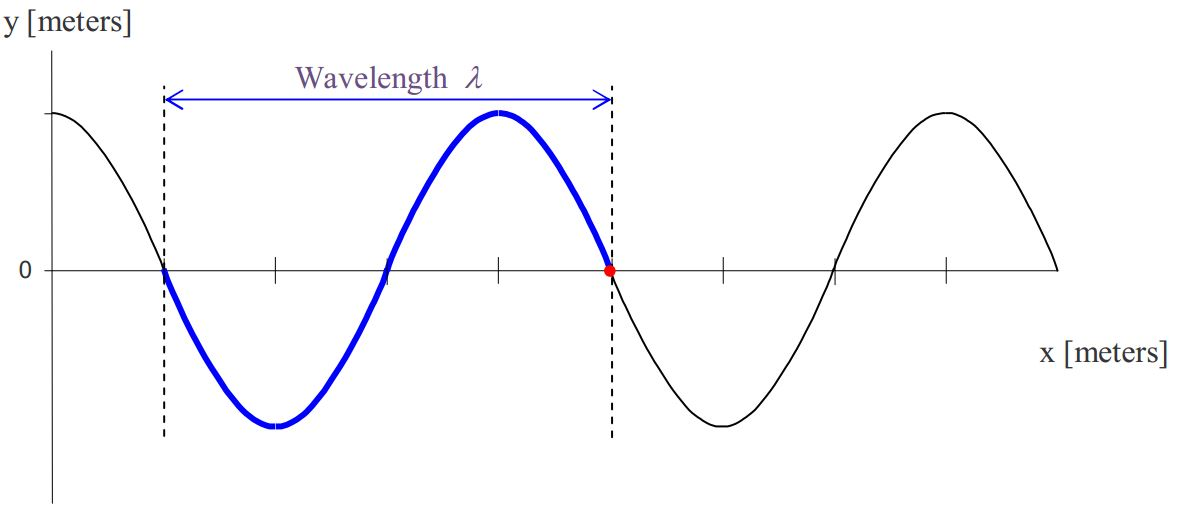Now, let’s allow some time to elapse, just enough time for the wave to move over one quarter of a wavelength.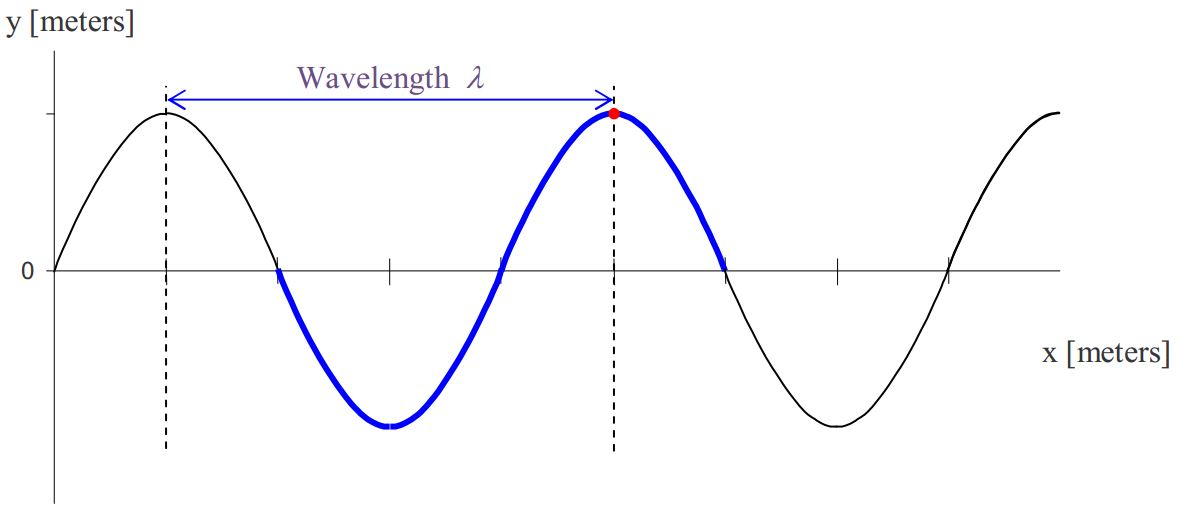In that time we note that the point on the string marked by the dot has moved from its equilibrium position to its maximum displacement from equilibrium position. As the wave has moved over one quarter of a wavelength, the point on the string has completed one quarter of an oscillation.

Let’s allow the same amount of time to elapse again, the time it takes for the wave to move over one quarter of a wavelength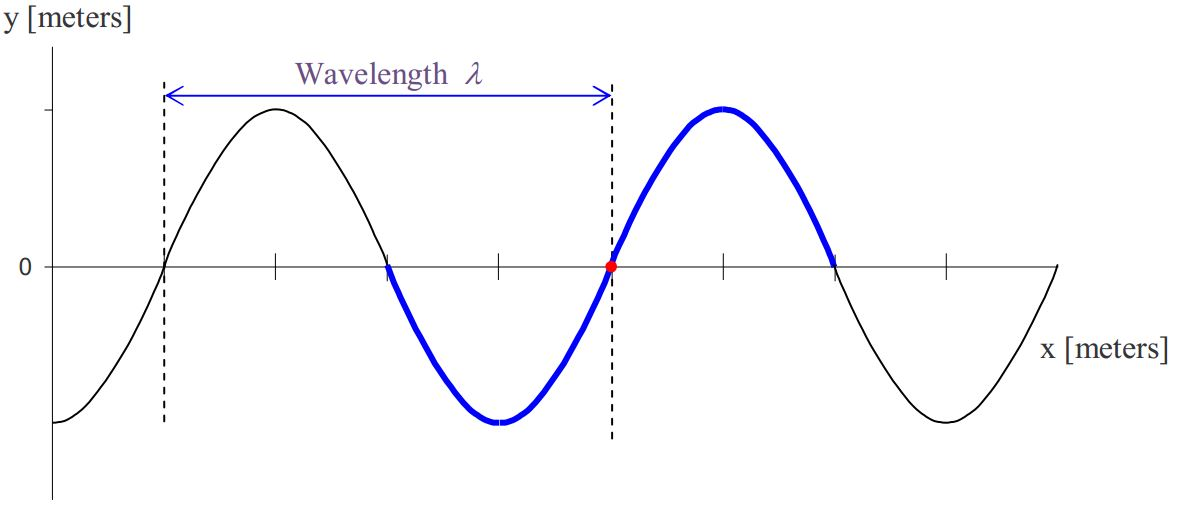At this point, the wave has moved a total of a half a wavelength over to the right, and the point on the string marked by the dot has moved from its equilibrium position up to its position of maximum positive displacement and back to its equilibrium position; that is to say, it has completed half of an oscillation.

Let’s let the same amount of time elapse again, enough time for the wave pattern to move over another quarter of a wavelength.The wave has moved over a total distance of three quarters of a wavelength and the point on the string that is marked with a dot has moved on to its maximum negative (downward) displacement from equilibrium meaning that it has completed three quarters of an oscillation.

Now we let the same amount of time elapse once more, the time it takes for the wave to move over one quarter of a wavelength.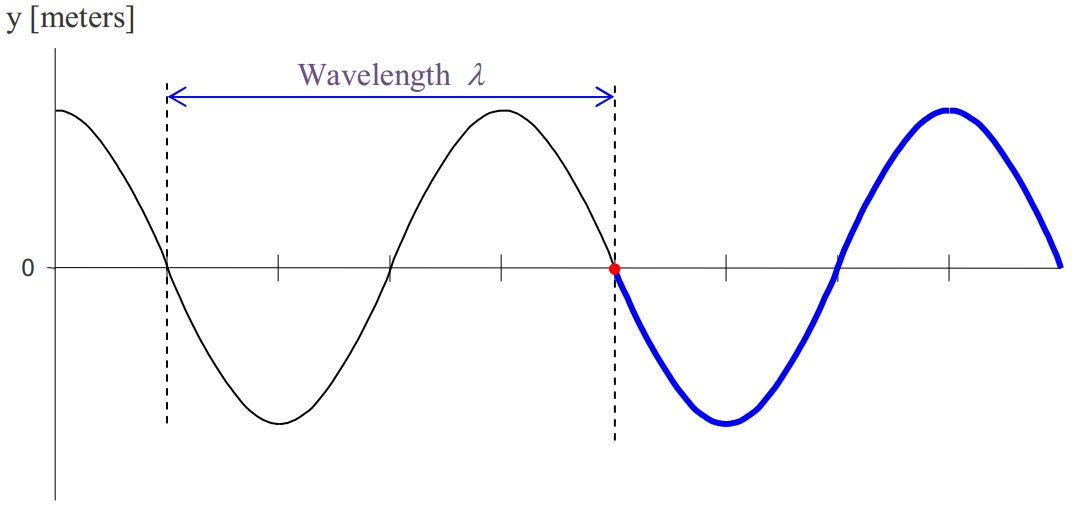At this point, the wave has moved over a distance equal to one wavelength and the point on the string marked by a dot has completed one oscillation. It is that point of the string whose motion we have been keeping track of that gives us a handle on the time. The amount of time that it takes for the point on the string to complete one oscillation is, by definition, the period of the wave. Now we know that the wave moves a distance of one wavelength $$\lambda$$ in a time interval equal to one period $$T$$. For something moving with a constant speed (zero acceleration), the speed is simply the distance traveled during a specified time interval divided by the duration of that time interval. So, we have, for the wave speed $$v$$ :

$v\space = \frac{\lambda}{T}\label{29-1}$

One typically sees the formula for the wave speed expressed as

$v\space=\lambda f\label{29-2}$

where the relation $$f\space=\frac{1}{T}$$ between frequency and period has been used to eliminate the period.

Equation 29-2 $$(\space v\space=\lambda f \space)$$ suggests that the wave speed depends on the frequency and the wavelength. This is not at all the case. Indeed, as far as the wavelength is concerned, it is the other way round—the oscillator that is causing the waves determines the frequency, and the corresponding wavelength depends on the wave speed. The wave speed is predetermined by the characteristics of the string—how taut it is, and how much mass is packed into each millimeter of it. Looking back on our discussion of how oscillations at one end of a taut string result in waves propagating through it, you can probably deduce that the greater the tension in the string, the faster the wave will move along the string. When the hand moves the end of the first segment up, the force exerted on the second segment of the string by the first segment will be greater, the greater the tension in the string. Hence the second segment will experience a greater acceleration. This goes for all the segments down the line. The greater the acceleration, the faster the segments pick up speed and the faster the disturbance, the wave, propagates along the string—that is, the greater the wave speed. We said that the wave speed also depends on the amount of mass packed into each millimeter of the string. This refers to the linear density $$\mu$$, the mass-per-length, of the string. The greater the mass-per-length, the greater the mass of each segment of the string and the less rapidly the velocity will be changing for a given force. Here we provide, with no proof, the formula for the speed of a wave in a string as a function of the string characteristics, tension $$F_{T}$$ and linear mass density $$\mu$$:

$v=\space \sqrt{\frac{F_{T}}{\mu}}\label{29-3}$

Note that this expression agrees with the conclusions that the greater the tension, the greater the wave speed; but the greater the linear mass density, the smaller the wave speed.

# Kinds of Waves

We’ve been talking about a wave in a string. Lots of other media, besides strings, support waves as well. You have probably heard, and probably heard of, sound waves in air. Sound waves travel through other gases and they travel through liquids and solids as well. Perhaps you have heard of seismic waves as well, the waves that travel through the earth when earthquakes occur. All of these waves fall into one of two categories. Which category is determined by the orientation of the lines along which the oscillations of the particles making up the medium occur relative to the direction of propagation of the waves. If the particles oscillate along lines that are perpendicular to the direction in which the wave travels, the wave is said to be a transverse wave (because transverse means perpendicular to). If the particles oscillate along a line that is along the direction in which the wave travels, the wave is said to be longitudinal. The wave in a horizontal string that we discussed at such length is an example of a transverse wave. Calling the direction in which the string extends away from the oscillator the forward direction, we discussed the case in which the particles making up the string were oscillating up and down. The wave travels along the string and the up and down directions are indeed perpendicular to the forward direction making it clear that we were dealing with transverse waves. It should be noted that the oscillations could have been from side to side or at any angle relative to the vertical as long as they were perpendicular to the string. Also, there is no stipulation that the string be horizontal in order for transverse waves to propagate in it. The string was said to be horizontal in our introduction to waves in order to simplify the discussion.

# Wave Power

Consider a very long stationary string extending from left to right. Consider a very short segment of the string, call it segment A, at an arbitrary distance along the string from the left end. Now suppose that someone is holding the left end of the long string in her hand and that she starts oscillating the left end of the string up and down. As you know, a wave will travel through the string from left to right. Before it gets to segment A, segment A is at rest. After the front end of the wave gets to segment A, segment A will be oscillating up and down like a mass on a spring. Segment A has mass and it has velocity throughout most of its motion, so clearly it has energy. It had none before the wave got there, so waves must have energy. By oscillating the end of the string the person has given energy to the string and that energy travels along the string in the form of a wave. Here we give an expression for the rate at which energy propagates along the string in terms of the string and wave properties. That rate is the energy-per-time passing through any point (through which the wave is traveling) in the string. It is the power of the wave.

The analysis that yields the expression for the rate at which the energy of waves in a string passes a point on the string, that is, the power of the wave, is straightforward but too lengthy to include here. The result is:

$P=\space 2\pi \sqrt{\mu F_{T}}\space f^2 \, y^2_{\max}$

As regards waves in a string with a given tension and linear mass density, we note that

$P\space \propto \, f^2 \, y^2_{\max}\label{29-4}$

This relation applies to all kinds of waves. The constant of proportionality depends on the kind of wave that you are dealing with, but the proportionality itself applies to all kinds of waves. I was reminded of this relation by a physical therapist who was using ultrasound waves to deposit energy into my back muscles. She mentioned that a doubling of the frequency of the ultrasonic waves would provide deeper penetration of the sound waves, but that it would also result in a quadrupling of the rate at which energy would be deposited in the tissue. Hence, to deposit the same total amount of energy that she deposited at a given frequency on one occasion; a treatment at double the frequency would either last one fourth as long (at the same amplitude) or would be carried out for the same amount of time at half the amplitude.

# Intensity

Consider a tiny buzzer, suspended in air by a string. Sound waves, caused by the buzzer, travel outward in all away-from-the-buzzer directions: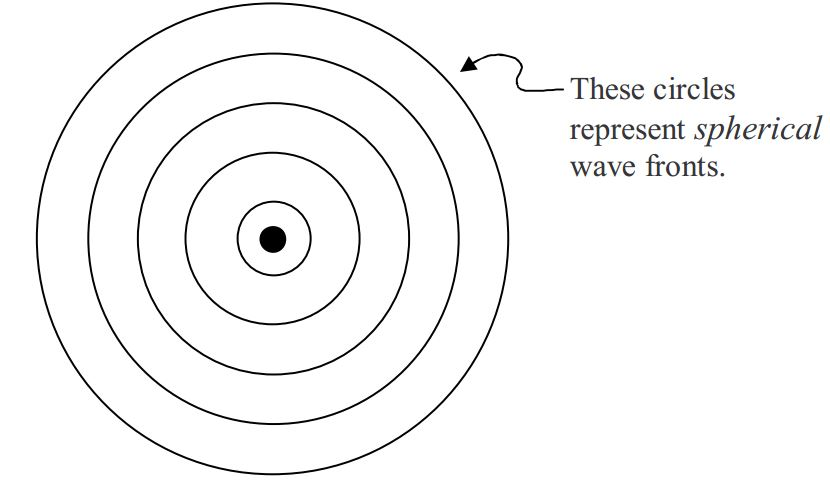In the diagram above, the black dot represents the buzzer, and the circles represent wavefronts—collections of points in space at which the air molecules are at their maximum displacement away from the buzzer. The wavefronts are actually spherical shells. In a 3D model of them, they would be well represented by soap bubbles, one inside the other, all sharing the same center. Note that subsequent to the instant depicted, the air molecules at the location of the wavefront will start moving back toward the buzzer, in their toward and away from the buzzer oscillations, whereas the wavefront itself will move steadily outward from the buzzer as the next layer of air molecules achieves its maximum displacement position and then the next, etc.

Now consider a fixed imaginary spherical shell centered on the buzzer. The power of the wave is the rate at which energy passes through that shell. As mentioned, the power obeys the relation

$P\space \propto \, f^2 \, y^2_{\max}$

Note that the power does not depend on the size of the spherical shell; all the energy delivered to the air by the buzzer must pass through any spherical shell centered on the buzzer. But the surface of a larger spherical shell is farther from the buzzer and our experience tells us that the further you are from the buzzer the less loud it sounds suggesting that the power delivered to our ear is smaller. So how can the power for a large spherical shell (with its surface far from the source) be the same as it is for a small spherical shell? We can say that as the energy moves away from the source, it spreads out. So by the time it reaches the larger spherical shell, the power passing through, say, any square millimeter of the larger spherical shell is relatively small, but the larger spherical shell has enough more square millimeters for the total power through it to be the same.

Now imagine somebody near the source with their eardrum facing the source.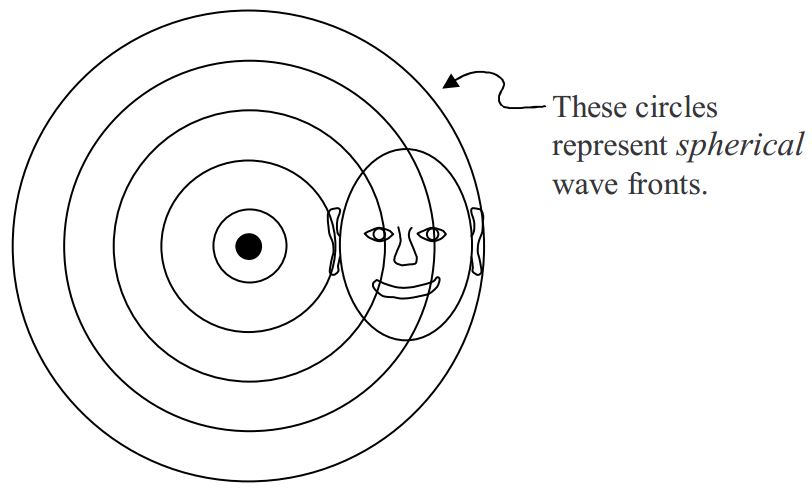The amount of energy delivered to the ear is the power-per-area passing through the imaginary source-centered spherical shell whose surface the ear is on, times the area of the eardrum. Since the spherical shell is small, meaning it has relatively little surface area, and all the power from the source must pass through that spherical shell, the power-per-area at the location of the ear must be relatively large. Multiply that by the fixed area of the eardrum and the power delivered to the eardrum is relatively large.

If the person is farther from the source,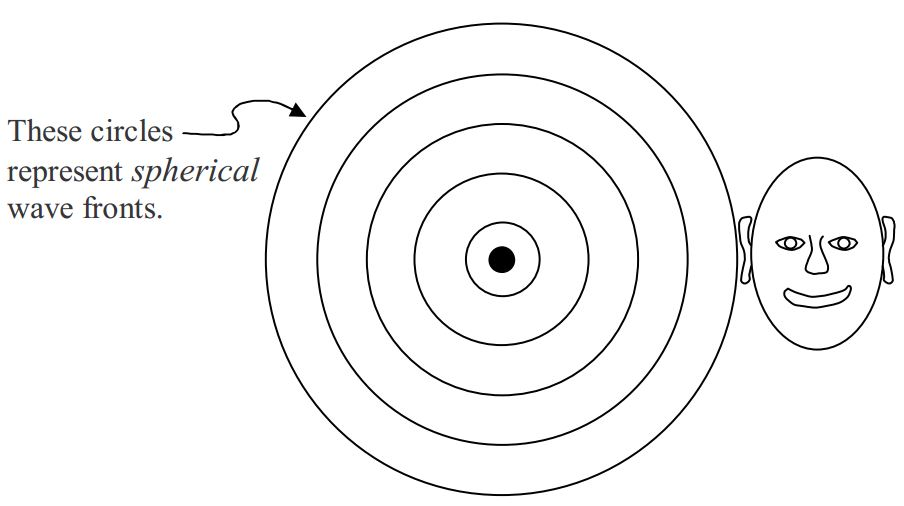the total power from the source is distributed over the surface of a larger spherical shell so the power-per-area is smaller. Multiply that by the fixed area of the eardrum to get the power delivered to the ear. It is clear that the power delivered to the ear will be smaller. The farther the ear is from the source; the smaller is the fraction of the total power of the source, received by the eardrum.

How loud the buzzer sounds to the person is determined by the power delivered to the ear, which, we have noted, depends on the power-per-area at the location of the ear.

The power-per-area of sound waves is given a name. It is called the $$intensity$$ of the sound. For waves in general (rather than just sound waves) we simply call it the intensity of the wave. While the concept of intensity applies to waves from any kind of source, it is particularly easy to calculate in the case of the small buzzer delivering energy uniformly in all directions. For any point in space, we create an imaginary spherical shell, through that point, centered on the buzzer. Then the intensity $$I$$ at the point (and at any other point on the spherical shell) is simply the power of the source divided by the area of the spherical shell:

$I=\frac{P}{4\pi \, r^2}\label{29-5}$

where the $$r$$ is the radius of the imaginary spherical shell, but more importantly, it is the distance of the point at which we wish to know the intensity, from the source. Since $$P\space \propto \, f^2 \, y^2_{\max}$$, we have

$I\space \propto \, \frac{f^2\space y^2_{\max}}{r^2}\label{29-6}$

At a fixed distance from a source of a fixed frequency, recognizing that $$y_{max}$$ is the amplitude of the waves we have

$I \, \propto \, (\mbox{Amplitude})^2\label{29-7}$